Math Concepts

# How to add Fractions with different combinations?

295 views

## Introduction

Fraction addition is a very important concept in mathematics. Mastering it in school is crucial as fractions are used heavily in further studies. This article covers the different types of fractions, the fraction addition, and the difficulties students face in handling fractions.

### How to add Fractions with different combinations?-PDF

This article covers the different types of fractions, the addition of fractions, and the difficulties students face while handling fractions. Here is a downloadable PDF to explore more.

## Why Do Students Struggle with Fractions?

Many teachers and parents know, learning the various fraction operations can be difficult for many children because of the gaps in the conceptual understanding of fractions. Just like the numbers, we use all the four arithmetic operations (+, -, x, ÷) with Fractions.

Students also need to know how to compare fractions and simplify them for easy calculations.  Without this basic knowledge about fractions, it would be difficult for the students to work on other topics from higher grades such as algebra, rational numbers, and so on.

Without these basics such as fractions, children tend to lose interest in math when they move to higher grades. They believe that math is simply “not for them” which can be the other way round when the basics are strong.

## What is a Fraction?

A Fraction is a part of something, like a group or a region. The upper part of a fraction is called a numerator and the lower part is called a denominator.

It is a ratio between two numbers. The sign for the fraction is ‘/’ or ‘̶’.

Fractions are represented as shown below: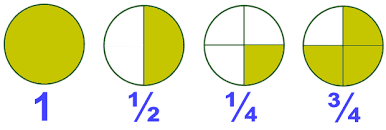Example: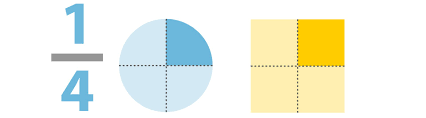1/4 is a Fraction. We read it as “One-Fourth”. Here 1 is the numerator and 4 is the denominator. The Numerator represents the number of equal parts that have been taken out. The denominator represents the total number of equal parts into which the whole has been divided.

## Types of Fractions

Proper Fractions

A Proper fraction is a fraction whose numerator is smaller than its denominator. The value of the proper fraction is always less than 1.

Examples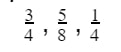Improper Fractions

An improper fraction is a fraction whose numerator is greater than or equal to its denominator.

Note: To go from improper fraction to mixed fraction, simply divide the Numerator by the Denominator. The Remainder over the Divisor is the fractional portion and the quotient is the number of wholes in the improper fraction.

Examples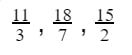Mixed Fractions

A mixed fraction is a combination of a whole and a fraction.

Note: Mixed fractions can be converted into an improper fraction by using the below formula

(DENOMINATOR  X WHOLE NUMBER + NUMERATOR)/DENOMINATOR

Examples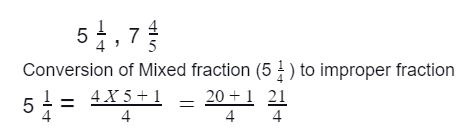## Fraction Addition with the Same Denominator (Like Fractions)

When fractions have the same denominators, we simply add the numerators as indicated and place the result over the common denominator.

Adding Proper Fractions with the Same Denominator

When adding the proper fractions with the same denominators (also called Like Fractions), only the numerators are added and the denominator remains the same. Always reduce your final answer to its lowest term.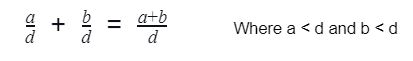Example: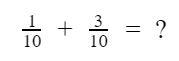Solution:

Step 1: Here denominators are the same, so just add numerators, that is 1 + 3 = 4

Step 2: Write the common denominator 10.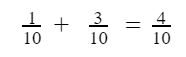Step 3: Reduce the fraction to get the lowest form. The lowest form is obtained by dividing both numerator and denominator with HCF of the two. Here, HCF of 4 and 10 is 2.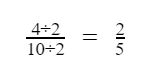Pictorial representation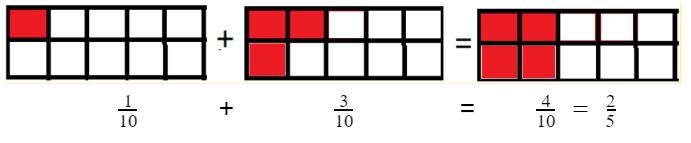Adding Improper Fractions with the Same Denominator

Similar to proper fractions, in the case of improper fractions too, only the numerators are added and the denominator remains the same. Always reduce your final answer to its lowest term.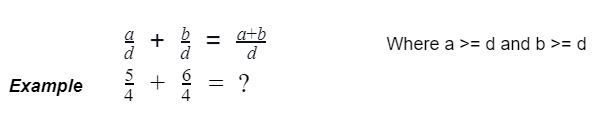Solution

Step 1: Here denominators are the same, so just add numerators, that is 5 + 6 = 11

Step 2: Write the common denominator 4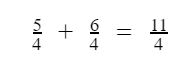Example with pictorial representation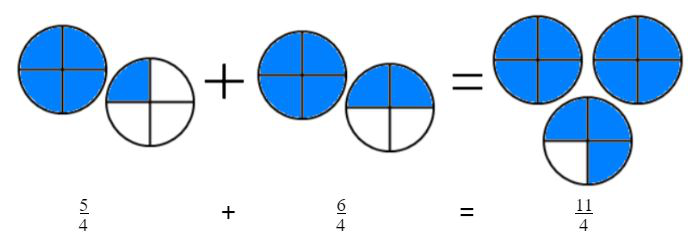Adding Mixed Fractions with the Same Denominator

There are two different methods to add mixed fractions.

Method 1: Add the whole numbers and fractions separately and then arrive at the final answer by combining the whole numbers and the fractions received after adding.

Method 2: First convert the mixed fraction to an improper fraction, then add the improper fractions together, simplify and convert the answer back to a mixed fraction.

Please watch the video below to understand how to add mixed Fractions with the same denominator:

## Adding Fractions with Different Denominators (Unlike Fractions)

For adding fractions with different denominators, we first need to make the fractions to be added as fractions with the same denominator. For this, we need to find LCM of two numbers and make the Unlike Fractions into Like Fractions.

Adding Proper Fractions with Different Denominator

When fractions have different denominators, the first step is to make them into equivalent fractions with the same denominators by using LCM. When all denominators are the same, simply add the numerators and place the result over the common denominator.

Example: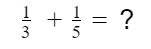Step 1: Denominators are different in the given fractions. Find the LCM of 3, 5 to make the same denominators. The LCM of 3 & 5 is 15

Step 2:  To make the denominators the same, multiply with 5 for the first fraction’s (1/3) numerator and denominator, then the first fraction becomes (5/15)

Step 3:  To make the denominators same multiply with 3 for the second  fraction’s (1/5) numerator and denominator, then the second fraction becomes (3/15)

Step 4:  Now denominators are the same, now simply add the numerators then copy the common denominator. Always reduce your final answer to its lowest term.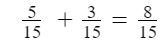Solution with pictorial representation: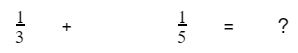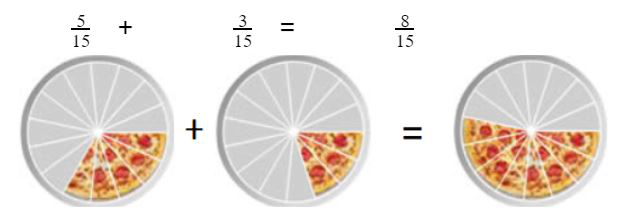Adding Improper Fractions with different denominator

When fractions have different denominators, the first step is to make them equivalent fractions with the same denominators by using LCM. When all denominators are the same, simply add the numerators and place the result over the common denominator.

Example: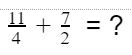Solution:

Step 1: Denominators are different in the given fractions. Find the LCM of 4 and 2 to make it into equivalent fractions with the same denominators. The LCM of 4 & 2 is 4

Step 2: Denominator of First fraction (11/4) is already 4 and it remains as is.

Step 3: To make denominators the same, we need to multiply the denominator of the second fraction (7/2) with 2 since we are multiplying the denominator with 2, the numerator should also be multiplied with 2 to make it into an equivalent fraction then the second fraction becomes 14/4

Step 4: Now denominators are the same, now simply add the numerators then copy the common denominator. Always reduce your final answer to its lowest term. In this example, we have converted the improper into a mixed fraction.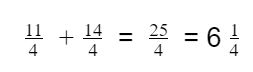Adding Mixed Fractions with Different Denominator

When mixed fractions have different denominators the first step is to convert mixed fractions to an improper fraction, then find the LCM for both the denominators. When all denominators are the same, simply add the numerators and place the result over the common denominator.

Example: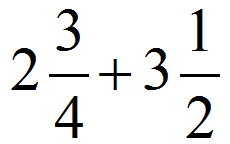Solution:

Step 1: Convert the mixed fractions to be added into improper fractions, then the fractions will be 11/4 and 7/2

Step 2: Since the Denominators are different in the given fractions, find the LCM of 4 & 2 to make them as equivalent fractions with the same denominators. The LCM of 4 & 2 is 4

Step 3:  Denominator of First fraction (11/4) is already 4, so the fraction remains as is.

Step 4: To make denominators the same, multiply both numerator and denominator by 2 for the second fraction,(7/2), then the second fraction becomes 14/4

Step 5:  Now denominators are the same, now simply add the numerators then copy the common denominator. Always reduce your final answer to its lowest term, wherever necessary.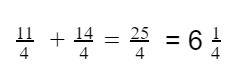Note: LCM (Least Common Multiple) is the smallest positive integer that is divisible by two or more given numbers

Here is the video explaining LCM using Cuemath methodology:

## Adding Fractions with Co-prime Denominator

Adding Proper Fractions with Co-prime Denominator

When fractions have co-prime denominators the first step is to find equivalent fractions so that all of the denominators are the same. When all denominators are the same, simply add the numerators and place the result over the common denominator.

Example: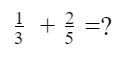Solution:

Step 1: Cross multiply numerator and denominator of the two fractions and add as shown below:

That is (1 x 5) + (2 x 3) = 11

Step 2: Multiply the two denominators together to get the denominator of the answer

That is 3 x 5 = 15

Adding Improper Fractions with Co-prime Denominator

When fractions have co-prime denominators the first step is to find equivalent fractions so that all the denominators are the same. When all denominators are the same, simply add the numerators and place the result over the common denominator.

Example: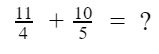Solution:

Step 1: To get Numerator value Multiply the numerator of each fraction by the denominator of the other

That is (11 x 5) + (10 x 4) = 95

Step 2: Multiply the two denominators together to get the denominator of the answer

That is 4 x 5 = 20

Step 3: Answer is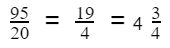(Improper fraction, converted to lowest form, which is then converted into a mixed fraction)

Adding Mixed Fractions with Co-prime Denominator

When fractions have co-prime denominators the first step is to find equivalent fractions so that all of the denominators are the same. When all denominators are the same, simply add the numerators and place the result over the common denominator.

Example: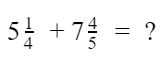Solution:

Step 1: Convert first and second fractions to improper fractions, then the fractions will be 21/4 and 39/5

Step 2: To get Numerator value Multiply the numerator of each fraction by the denominator of the other

That is (21 x 5) + (39 x 4) = 261

Step 3: Multiply the two denominators together to get the denominator of the answer

That is 4 x 5 = 20

Step 4: Answer is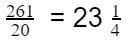## Summary

• A Proper Fraction has a numerator that is smaller than its denominator and represents a quantity that is less than 1

• An Improper Fraction has a numerator larger than its denominator and represents a quantity greater than 1 or equals to any whole more than 1

• Mixed Fraction is Greater than 1

• Any fraction with a denominator of 1 is equal to its numerator.

• Division by zero is Undefined, so the Denominator of a fraction can never be zero.

• Fraction addition can be calculated by different methods for the different types of fractions according to whether the denominator is the same or not.

• HCF and LCM are used to find the equivalent fractions and also in converting the, unlike fractions into like fractions. In the below video, Cuemath students explain HCF and LCM:

Cuemath, a student-friendly mathematics and coding platform, conducts regular Online Live Classes for academics and skill-development, and their Mental Math App, on both iOS and Android, is a one-stop solution for kids to develop multiple skills.Understand the Cuemath Fee structure and sign up for a free trial.

## 1. How to find HCF?

HCF stands for Highest Common Factor. We calculate the factors of both the given numbers. The highest factor which divides them both equally is the HCF. You can read more about it here.

## 2. How to find LCM?

LCM stands for Lowest Common Multiple. We find the multiples of both numbers and then see the lowest multiple they have in common. This gives us the LCM. You can read more about it here.

## 3. How to add fractions?

Fraction Addition has different methods for different type of fractions. For fractions with same denominators, just add both the numerators and keep the denominator as same. The fraction addition of fractions with different denominators is a bit complicated. We suggest you to follow the method explained above in the article.